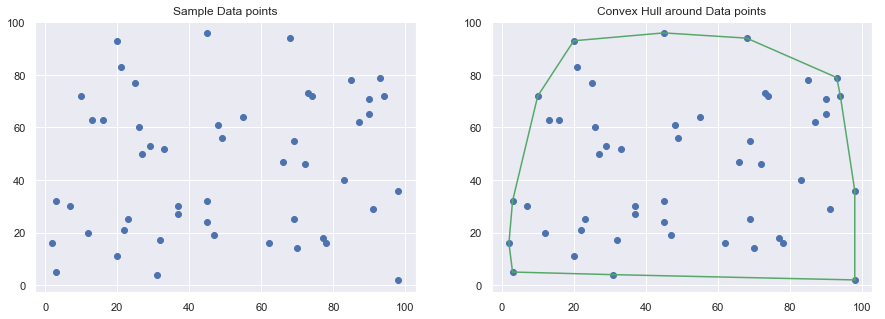# Understanding Graham scan algorithm for finding the Convex hull of a set of Points

Convex Hull is one of the fundamental algorithms in Computational geometry used in many computer vision applications like Collision avoidance in Self Driving Cars, Shape analysis and Hand Gesture-recognition, etc.

By Definition, A Convex Hull is the smallest convex set that encloses a given set of points. For Example, Given a set of points P in 2D or 3D space, a subset of points in P which fully encloses all points is called the Convex Hull. Take a look at the below figure.There are a number of algorithms proposed for computing the convex hull of a finite set of points with various computational complexities. One such algorithm is the Graham Scan algorithm with a worst case complexity of O(nlogn) which is going to be the topic of my discussion in this post.

Before we get into the algorithm we must understand a few basics upon which the Graham scan is built upon because once you understand them, convex hull would become fairly easy to implement.

## Convex vs Concave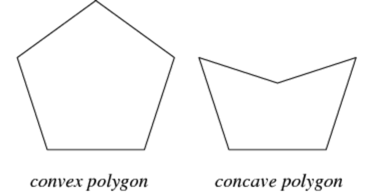Every polygon is either Convex or Concave. A polygon is said to be Convex if all its internal angles are less than $$180^{\circ}$$ as you can see in the figure above. It’s Concave if the polygon has angles greater than $$180^{\circ}$$.

There is another way to define a Convex Polygon. If every point on every line segment between two points inside or on the boundary of the polygon remains inside or on the boundary then the polygon is said to be Convex. Take a look at the below image to understand the above statement.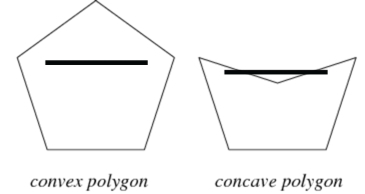## Counterclockwise turns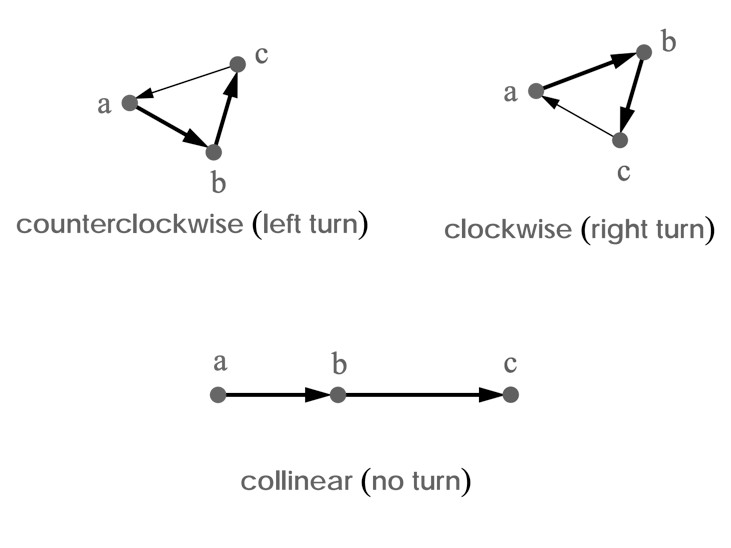There are many ways to determine if the tuple of 3 points forms a clockwise turn or a counterclockwise turn or if they are collinear. One of the ways is by finding the determinant of the matrix formed by the three points. If the determinant is positive, then a->b->c is counterclockwise; if the determinant is negative, then a->b->c is clockwise; if the determinant is zero then a->b->c are collinear.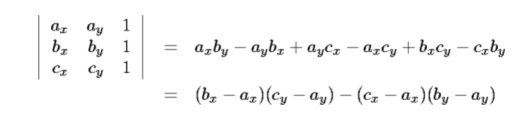The determinant is not the most efficient way to identify the turn because of the complexity of multiplication and addition operations. There is a more efficient way which uses the slope of the lines formed by the points.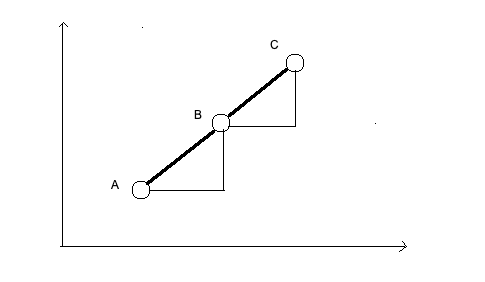Slope of line segment (A, B): σ = (y2 – y1)/(x2 – x1)
Slope of line segment (B, C): τ = (y3 – y2)/(x3 – x2)

If σ > τ, the orientation is clockwise (right turn)

Using above values of σ and τ, we can conclude that,
the orientation depends on sign of below expression:

(y2 – y1)*(x3 – x2) – (y3 – y2)*(x2 – x1)

The above expression is negative when σ < τ, i.e., counterclockwise

The python code we will be using later on for determining the CCW is as below:

def ccw(a, b, c):
return (b - a) * (c - a) - (c - a) * (b - a)

## Polar Angle

In the plane, the polar angle theta is the counterclockwise angle from the x-axis at which a point in the xy-plane lies.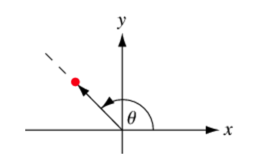We use a special function in math library atan2 to find the angle in radians.

from math import atan2

def polar_angle(p0, p1):
y_span=p0-p1
x_span=p0-p1
return atan2(y_span,x_span)

## Graham Scan Algorithm

With the basics in place, we are ready to understand the Graham Scan Convex Hull algorithm. The steps in the algorithm are:

1. Given a set of points on the plane, find a point with the lowest Y coordinate value, if there are more than one, then select the one with the lower X coordinate value. Call this point an Anchor point.
2. Sort all the points based on the polar angle they make with the anchor point. If two points make the same angle with Anchor Point P, then sort it by distance from P
3. Initialize the convex hull array with the anchor point and the first element in the sorted array.
4. Iterate over each point in the sorted array and see if traversing to a point from the previous two points makes a clockwise or a counter-clockwise direction. If clockwise then reject the point and move on to the next point. Continue this till the end of the sorted array.

Take a look at the below simulation to understand the Graham Scan process.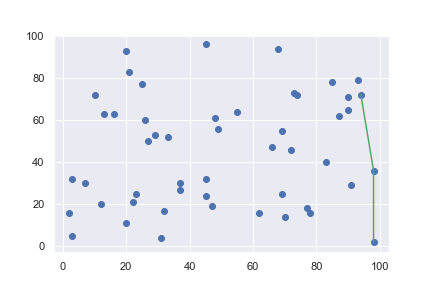The complete notebook with the steps is given here.

https://github.com/muthuspark/ml_research/blob/master/Construction%20of%20Convex%20Hull%20using%20Graham%20Scan.ipynb

#### Step 1: Given a set of points on the plane, find a point with the lowest Y coordinate value, if there are more than one, then select the one with the lower X coordinate value. Call this point an Anchor point.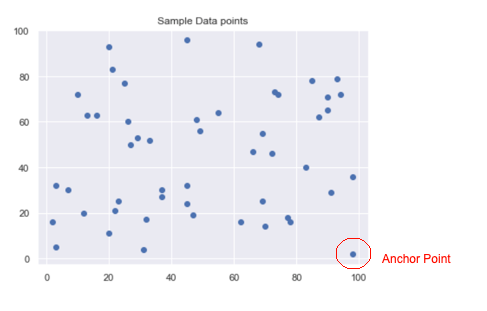anchor_point = datapoints
for _, point in enumerate(datapoints):
if point < anchor_point:
anchor_point = point
elif point == anchor_point and point < anchor_point:
anchor_point = point
print(anchor_point)

#### Step 2: Sort all the points based on the polar angle they make with the anchor point. If two points make the same angle with Anchor Point P, then sort it by distance from P

from math import atan2

def polar_angle(p0, p1):
y_span=p0-p1
x_span=p0-p1
return atan2(y_span,x_span)

# find the angle
datapoints_angles = []
origin = [0,0]
for _, point in enumerate(datapoints):
datapoints_angles.append([point,point, polar_angle(anchor_point, point)])

datapoints_angles = np.array(datapoints_angles)
datapoints_angles = datapoints_angles[datapoints_angles[:,2].argsort()]
sorted_datapoints =  datapoints_angles[:,(0,1)]

#### Step 3: Initialize the convex hull array with the anchor point and the first element in the sorted array.

convex_hull = [anchor_point, sorted_datapoints]

#### Step 4: Iterate over each point in the sorted array and see if traversing to a point from the previous two points makes a clockwise or a counter-clockwise direction. If clockwise then reject the point and move on to the next point. Continue this till the end of the sorted array.

def ccw(a, b, c):
return (b - a) * (c - a) - (c - a) * (b - a)

for point in sorted_datapoints[1:]:
while ccw(convex_hull[-2],convex_hull[-1], point)<=0:
del convex_hull[-1] # backtrack
convex_hull.append(point)

That’s all!

PS: Code I wrote for sorting the array at step 2 doesn’t sort the array if there are duplicate angles. I have to update the code.

References:

1. https://en.wikipedia.org/wiki/Convex_hull_algorithms
2. http://mathworld.wolfram.com/PolarAngle.html